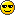Lost universe of ProgramingUSERNAME :- Forum Post:-114Lost universe of Programing :: --=| SOURCE CODE |=-- :: VB 6.0 Code Share

# Array basics for beginnersAuthorMessageNumber of posts : 37
Age : 33
Location : Lucknow
Job/hobbies : Software Engeener
What U like To do ? : I Rocks With Computer System.
Registration date : 2008-01-09Subject: Array basics for beginners5/8/2008, 1:42 am ' There are two areas where arrays can be declared.' Firstly in the General Declarations section. Arrays declared' here are seen by all procedures in thi ' s form or module:Private arrayName([intLow To] intHigh) As dataType' For example:Private lngArray(2 To 4) As Long' You can also declare the array as Public, making it visible to' all forms and modules in the applicati ' on:Public arrayName([intLow To] intHigh) As dataType' For example:Public strArray(0 To 200) As String' Secondly, within procedures:Dim arrayName([intLow To] intHigh) As dataType' For example:Dim intArray(0 To 9) As Integer' You can also specify element size like a string variable:Dim arrayName([intLow To] intHigh) As dataType * intByteSize' For example:Dim strArray(3 To 14) As String * 256' Also, you can just specify the array length by omitting the' [intLow To] code shown above. This spe ' cifies the upper' element's index, not neccessarily the ' arrays length:Dim ArrayName(intHigh) As dataType' For example:Dim intArray(9) As Integer' This array will be indexed from 0 by default (so the array's' size will be one greater than intHigh) ' unless the following' line is added to the General Declarati ' ons section:Option Base 1' You can also do this (for single dimensional arrays only):Dim scores As Variantscores = Array(81, 49, 80, 71, 92, 66)' The above scores array will be indexed from 0 unless Option Base 1' is added to the General Declarations s ' ection.' VB supports static and dynamic arrays. Static arrays are fixed in' size and can't be changed at runtime, ' dynamic array sizes can.' Static arrays are more memory efficient:Dim array2(10 To 20) As Integer' Dynamic arrays do not have a size defined when initialized:Dim array3() As Integer' You must ReDim a dynamic array to change its size at runtime:ReDim array3(1 To 4) As Integer' You can preserve the contents of the array elements when you' ReDim the array by using the Preserve ' keyword with ReDim:ReDim Preserve array3(1 To 9) As Integer' You can also assign to a dynamic array directly from another' array without specifying size:Dim array4() As Integerarray4 = array3' array4 will now be initialize with the size' (and element values if any) of array3. ' ' Visual Basic allows you to use For Each ... Next to enumerate' the items in an array:Dim element As VariantFor Each element In array4()'code to process array elements sequentiallyNext' Because arrays do not have a Count property you can use the' UBound and LBound methods to establish ' its length.' You could use a loop like the following:For x = LBound(ArrayName()) To UBound(ArrayName())'code to process array items sequentiallyNext' The arrays empty parentheses are not required, so the first' line of code above could be like this: ' For x = LBound(ArrayName) To UBound(ArrayName)' To establish the length you could also use the following code' that subtracts the LowerBound value fr ' om the UpperBound value,' then adds one to the result because th ' e array elements include' both upper and lower, then returns the ' result As Integer:Function GetCount(AnyArray As Variant) As IntegerOn Error Resume NextDim length As Integerlength = UBound(AnyArray) - LBound(AnyArray) + 1GetCount = length ' + 1 = inclusiveEnd Function' If you know the data type of the array you could declare' the AnyArray argument as that type ins ' tead of As Variant' to improve performance:Function GetCount(sngArray() As Single) As IntegerOn Error Resume NextDim length As Integerlength = UBound(sngArray) - LBound(sngArray) + 1GetCount = length ' + 1 = inclusiveEnd Function' Calling the function is as easy as:intCount = GetCount(myArray())' Multi-dimensional arrays' Each dimension of the array must contain the same data type.' The second-last and last dimensions of a multi-dimensional array' are normally considered to be a Row an ' d a Column respectively.Private multiArray(1 To 5, 1 To 3) As Integer' So for a two dimensional array in VB the dimension (row) is' defined first, and the number of eleme ' nts (cols) for each' dimension defined second:Public 2DArray(1 To 2, 1 ToAs Long' This array is a two dimensional array containing 8 elements' in each dimension:Dim i1 As Integer, i2 As IntegerFor i1 = 1 To 2For i2 = 1 To 82DArray(i1, i2) = "Cell " & CStr(i1) & "," & CStr(i2)NextNext' You can also assign arrays to the elements of other arrays to' create multi-dimensional arrays:Public Sub CreateMultiArray()Dim intX As Integer ' Declare counter variable' Declare and populate an integer arrayDim countersA(1 To 4) As IntegerFor intX = 1 To 4countersA(intX) = intXNext intX' Declare and populate a string arrayDim countersB(1 To 4) As StringFor intX = 1 To 4countersB(intX) = "hello"Next intX' Declare a new two-member arrayDim arrX(1 To 2) As Variant' Populate the array with other arraysarrX(1) = countersA()arrX(2) = countersB()' Display a member of each arrayMsgBox arrX(1)(2)MsgBox arrX(2)(3)End Sub' To increase the size of an array without losing its current' values use the Preserve keyword:_________________""»-(¯`v´¯)-»"Er Amit Tripathi"»-(¯`v´¯)-»"Array basics for beginnersPage 1 of 1
 Similar topics» RUST's Beginners Guide & Tips and Tricks
» Neuer beginner Clan Apocalyptic Vendetta!
» The basics of winning chess
» Phaser Banks, Phaser Arrays, and Phaser Strips
» Arena - beginners question

Permissions in this forum:You cannot reply to topics in this forumJump to: Select a forum||--WRITE YOUR PROBLEM IN ANY TECHENOLOGY|----=| SOURCE CODE |=--|   |--C/C++ SOURCE CODE|   |--CODE FOR C# & ASP.NET|   |--CODE FOR JAVA|   |--DATA BASE MANAGEMENT|   |--VB 6.0 Code|   |--VB.NET CODE|   |--Java Script and Html|   |----=| TUTORIALS |=--|   |--TUTORIALS FOR MESSENGER|   |--JAVA|   |--ETHICAL HACKING & NETWORKING|   |--TOOL & TIPS|   |--MOBILE RELATED|   |----=|WINDOWS|=--    |--WINDOW VISTA Problem    |--WINDOW XP Problem    |--Software Discription